Question

# Three balls are projected at the same speed from a cliff. Ball 1 is fired horizontally...

Three balls are projected at the same speed from a cliff. Ball 1 is fired horizontally and hits the ground at t1. Ball 2 is fired at an angle of 30 degrees above the horizontal plane and hits the ground at t2. Ball 3 is fired at an angle of 60 degrees above the horizontal plane and hits the ground at t3. Which ball hit the ground from least to greatest. Which equation would you use to calculate the distance traveled by each ball?

A. t3<t2<t1

B. t1<t2=t3

C. t1=t2=t3

D. t1<t3<t2

E. t1<t2<t3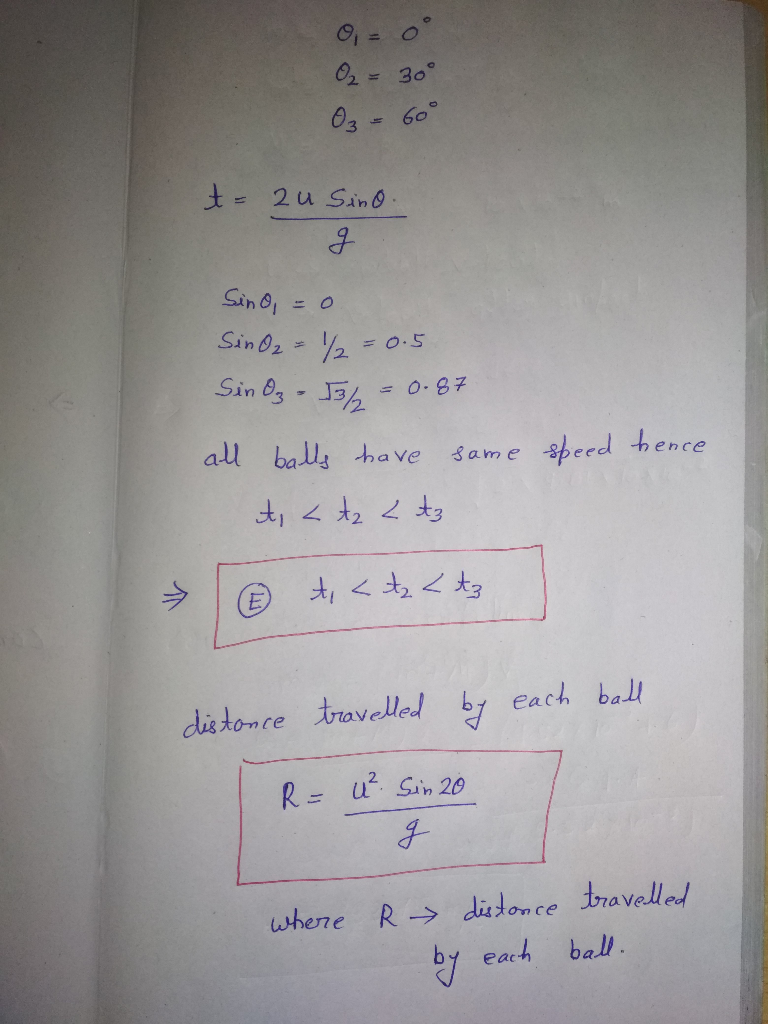#### Earn Coins

Coins can be redeemed for fabulous gifts.

Similar Homework Help Questions
• ### A ball is thrown horizontally of a cliff. If the initial speed of the ball is...

A ball is thrown horizontally of a cliff. If the initial speed of the ball is (15.0+A) m/s and the cliff is (25.0+B) m high, how far from the base of the cliff will the ball land in the water below? Calculate the answer in meters (m) and rounded to three significant figures. A=8 B=58 A water balloon is launched at a speed of (15.0+A) m/s and an angle of 36 degrees above the horizontal. The water balloon hits a...

• ### A cannon tilted at 25" above the horizontal is fired from the top of a cliff...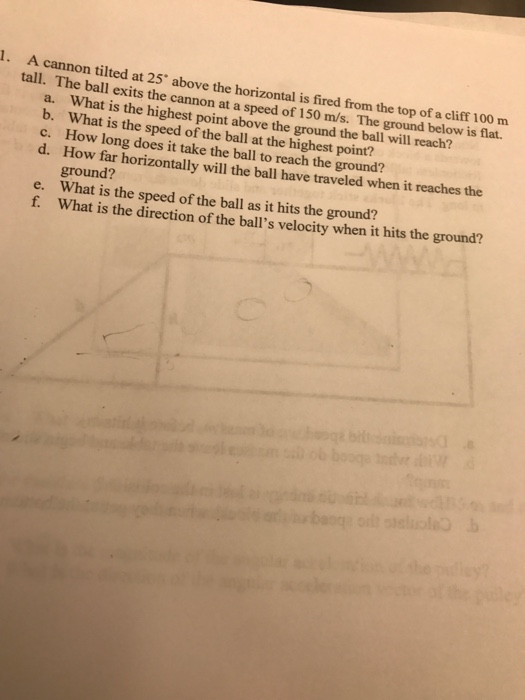A cannon tilted at 25" above the horizontal is fired from the top of a cliff 100 m exits the cannon at a speed of 150 m/s. The ground below is flat. tall. The ball Wh at is the highest point above the ground the ball will reach? What is the speed of the ball at the highest point? How long does it take the ball to reach the ground? How far horizontally will the ball have traveled when it...

• ### A baseball is projected horizontally with an initial speed of 12.9 m/s from a height of...

A baseball is projected horizontally with an initial speed of 12.9 m/s from a height of 2.35 m. At what horizontal distance will the ball hit the ground? (Neglect air friction.) What is the speed of the baseball when it hits the ground?

• ### 1) A projectile is fired horizontally from the top of a cliff as shown: 1.00 km...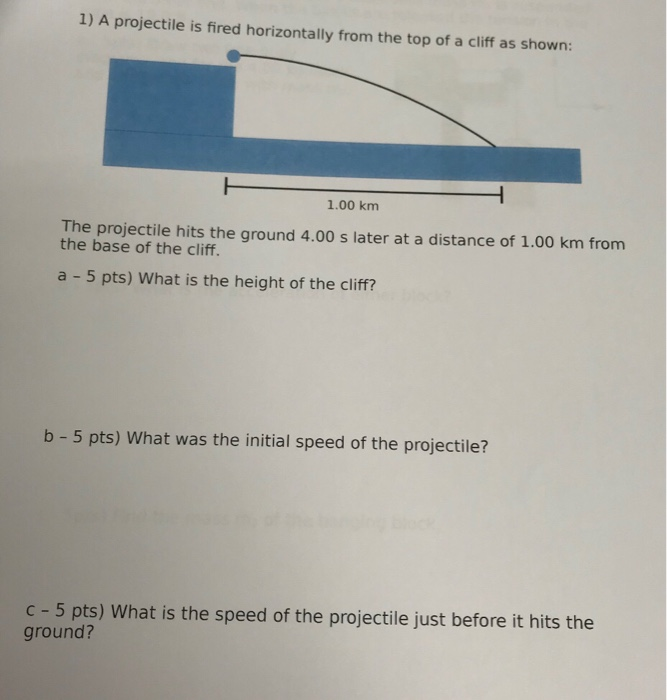1) A projectile is fired horizontally from the top of a cliff as shown: 1.00 km The projectile hits the ground 4.00 s later at a distance of 1.00 km from the base of the cliff. a 5 pts) What is the height of the cliff? b - 5 pts) What was the initial speed of the projectile? c - 5 pts) What is the speed of the projectile just before it hits the ground?

• ### A baseball is projected horizontally with an initial speed of 17.5 m/s from a height of...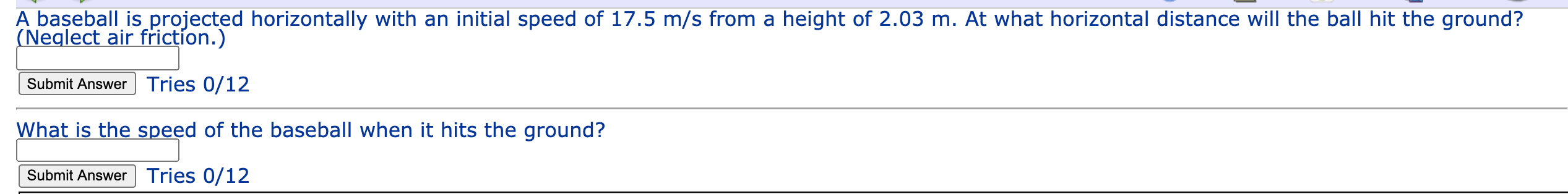A baseball is projected horizontally with an initial speed of 17.5 m/s from a height of 2.03 m. At what horizontal distance will the ball hit the ground? (Neglect air friction.) Submit Answer Tries 0/12 What is the speed of the baseball when it hits the ground? Submit Answer Tries 0/12

• ### help 1. A cannon tilted at 25" above the horizontal is fired from the top of...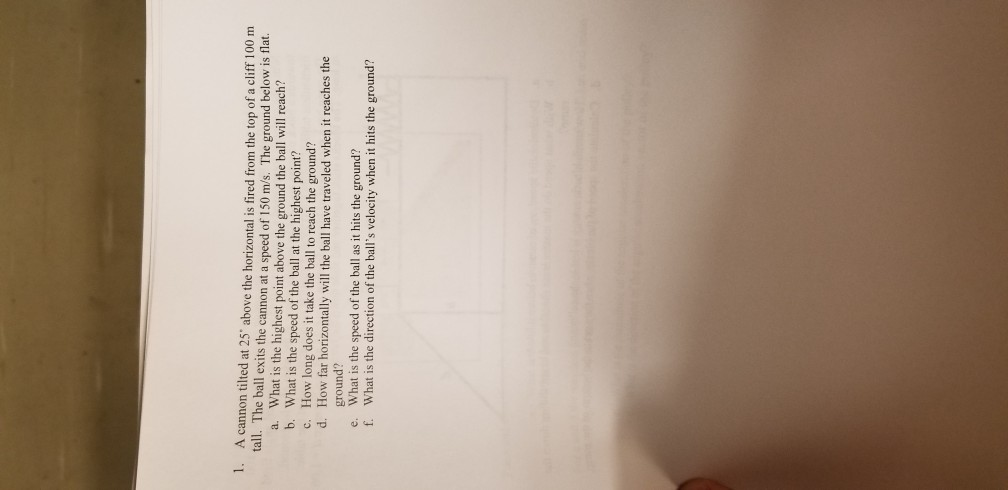help 1. A cannon tilted at 25" above the horizontal is fired from the top of a cliff 100 m tall. The ball exits the cannon at a speed of 150 m/s. The ground below is flat. What is the highest point above the ground the ball will reach? What is the speed of the ball at the highest point? How long does it take the ball to reach the ground? How far horizontally will the ball have traveled when...

• ### A cannonball is fired horizontally from the top of a cliff. The cannon is at height H = 70.0m above ground level, and t...

A cannonball is fired horizontally from the top of a cliff. The cannon is at height H = 70.0m above ground level, and the ball is fired with initial horizontal speed v0. Assume acceleration due to gravity to be g = 9.80m/s2 . Part A Assume that the cannon is fired at time t=0 and that the cannonball hits the ground at time tg. What is the y position of the cannonball at the time tg/2? Answer is 52.5 Part...

• ### 1. 2. Numerical Problems An aeroplane is flying horizontally at a speed of 100 m's releases...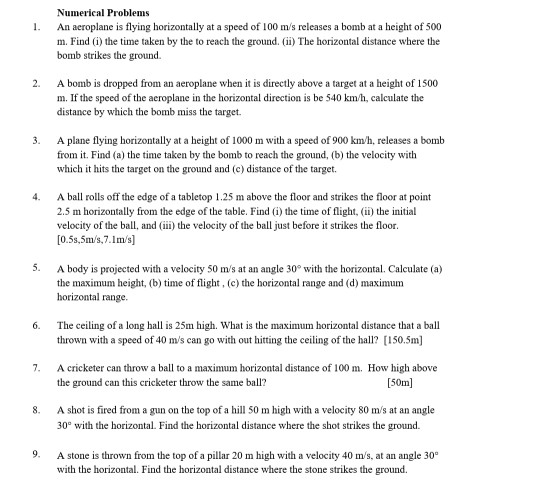1. 2. Numerical Problems An aeroplane is flying horizontally at a speed of 100 m's releases a bomb at a height of 500 m. Find (1) the time taken by the to reach the ground. (ii) The horizontal distance where the bomb strikes the ground A bomb is dropped from an aeroplane when it is directly above a target at a height of 1500 m. If the speed of the aeroplane in the horizontal direction is be 540 km/h, calculate...

• ### 6. Someone kicks a ball off of a 15-m-high cliff. The kick is at an angle...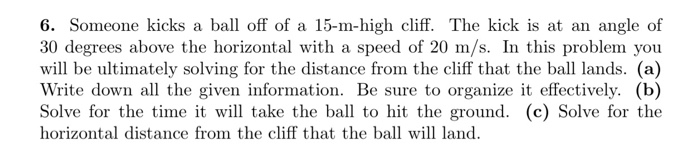6. Someone kicks a ball off of a 15-m-high cliff. The kick is at an angle of 30 degrees above the horizontal with a speed of 20 m/s. In this problem you will be ultimately solving for the distance from the cliff that the ball lands. (a) Write down all the given information. Be sure to organize it effectively. (b) Solve for the time it will take the ball to hit the ground. (c) Solve for the horizontal distance from...

• ### A cannon-ball is fired horizontally with a speed vo = 60.0 m/s above the edge of...

A cannon-ball is fired horizontally with a speed vo = 60.0 m/s above the edge of a cliff at point O a height h = 200 m directly above point B on the valley floor. Calculate the following: a. (4) The time t it takes the cannon ball to hit the valley floor at point C. b. (4) The horizontal range R . c. (6) The x- and y-components x v and y v , respectively, of the cannonball velocity...